# Algorithms

ARX implements a novel globally-optimal anonymization algorithm, Flash, which constructs a search space and determines the transformation with minimal information loss. Additionally, the complete solution space is classified, allowing users the explore potential alternative solutions to their anonymization problem. The Flash algorithm traverses the generalization lattice in a bottom-up breadth-first manner and constantly generates paths which branch like lightning flashes. It is based upon the following key ideas:

• Predictive tagging can be best exploited if the lattice is traversed vertically and in a binary fashion.
• When traversing a lattice vertically, execution time becomes volatile in terms of the representation of the input dataset (e.g., the order of the columns). This must be prevented by implementing a stable strategy.
• In order to achieve maximal performance, the algorithm should prefer checking transformations that allow the application of multiple optimizations.

### 1. Basic algorithm

Flash iterates over all levels in the lattice, starting at level 0. It enumerates all transformations on each level and calls findPath(node) if a transformation has not been tagged already. This method implements a greedy depth-first search towards the top node. The search terminates when either the top node is reached or the current node does not have a successor that is not already tagged.

```PriorityQueue pqueue = new PriorityQueue();
for (int i = 0; i < lattice.height; i++) {
for (Node node : sort(level[i])) {
if (!node.isTagged()) {
while (!pqueue.isEmpty()) {
}
}
}
}
}
```

When a path has been built, checkPath(path, pqueue) is called on it. It implements a binary search strategy. It starts by checking the node at position floor(1/2*(path.size-1)). Whenever a node is checked for anonymity, the algorithm also applies predictive tagging within the whole generalization lattice. Depending on the result of the check, it then proceeds with the lower or upper half of the path. Whenever a node is explicitly checked and determined to be non-anonymous, it is added to a priority queue. If a node is explicitly checked and determined to be anonymous, a reference to it is stored, as it could be the local optimum. There is no need to check whether a node has already been tagged, because findpath(node) always returns a path of untagged nodes. Furthermore, predictive tagging is always applied in the direction opposite to the one taken by the algorithm. Another important thing to note is that after the search terminates, the variable optimum will always hold a reference to the optimal anonymous node on the path (if there is any). The globally optimal node is determined by comparing the current local optimum with the current global optimum.

```private void findPath(Node node){
List path = new List();
for(Node up : sort(node.getSuccessors())){
if (!up.tagged){
node = up;
break;
}
}
}
return path;
}
```

After a path has been checked, the nodes in the priority queue are utilized to build new paths starting from the successors of the non-anonymous nodes that have been explicitly checked when processing the previous path. The general idea behind this is that these paths increase the potential to apply the roll-up and snapshot optimizations. A somewhat simplified description is that, the priority queue returns the nodes according to their level in the lattice. The algorithm starts with the minimal element, as this potentially increases the length of the created path. This, in turn, increases the potential to apply predictive tagging to other nodes in the lattice.

```private void check(List path, PriorityQueue pqueue) {
int low = 0; int high = path.size() - 1;
while (low <= high) {
int mid = (low + high) / 2;
Node node = path.get(mid);
if (!node.isTagged()) {
checkAndTag(node);
if (!node.isAnonymous()) {
for (final Node up : node.getSuccessors()) {
if (!up.isTagged()) {
}
}
}
}
if (node.isAnonymous()) high = mid - 1;
else low = mid + 1;
}
}
```

In case of an empty queue, the algorithm proceeds with the outer loop. It terminates when the outer loop terminates. The Flash algorithm further implements a general strategy that induces a total order on all nodes in the lattice. As indicated by the method sort() in the pseudocode, the algorithm follows this order whenever ititerates over a set of nodes.

### 2. Traversal strategy

An important property of an algorithm that implements a vertical traversal strategy is stability in terms of execution time. This is due to the fact that the order in which nodes are enumerated influences the order in which they are checked. In combination with a vertical strategy and predictive tagging, this leads to different lattice traversals, which leads to a varying number of anonymity checks. This finally leads to differences in the algorithm’s execution times. In practice, these differences can be, for example, triggered by changing the order of the columns in the input dataset. The solution to this problem is to apply a strategy that is independent of the representation of the input dataset whenever nodes are enumerated. The idea behind the strategy implemented by the Flash algorithm is to prefer nodes with a lower degree of generalization.

The algorithm utilizes three criteria for each node n. The criterion c1(n) returns the level of the node in the lattice:The criterion c2(n) is used to differentiate between nodes on the same level and resembles the Precision metric. It returns the average generalization over all quasi-identifiers: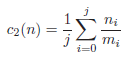Finally, the criterion c3(n) is utilized to differentiate between nodes on the same level which also result in the same average generalization. It represents the average over the number of distinct values on the current level of each quasi-identifier:These three criteria are then combined into a vector in the following way: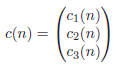A set of nodes is always traversed (by calling sort())  according to the totally ordered vector space induced by the relation <=, which defines the lexicographical order, i.e. c(n1) <= c(n2) iff: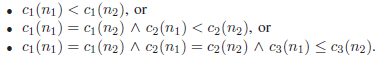The algorithm applies this order when enumerating nodes on a level and when enumerating successors of a node. Finally, the criterion also serves as the key for the entries in the priority queue. The strategy has to be implemented carefully. Sorting all levels in the lattice and all pointers to successors prior to the execution of the algorithm is too expensive. The algorithm therefore evaluates the priority function lazily and sort a nodes’ successors only when needed. Analogously, a level is sorted directly before iterating over it. This allows exclusion of all nodes which have already been tagged from the sorting step.

### 3. Example

The first two iterations of the Flash algorithm can be seen in the following figure: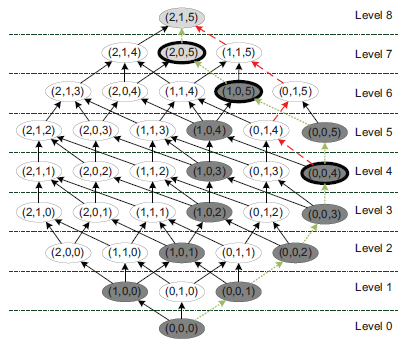It starts by building a path from the bottom node (0,0,0) to the top node (2,1,5). This results in the rightmost path, indicated by the dotted lines. This path is then checked in a binary manner, which results in explicit checks of the nodes (0,0,4), (1,0,5) (non-anonymous) and (2,0,5) (anonymous). The anonymity property of the other non-anonymous nodes (dark gray) is determined by applying predictive tagging from (0,0,4) and (1,0,5). The top-node is predictively tagged as being anonymous from the node (2,0,5). After checking the first path, the priority queue contains the nodes (1,0,5) and (0,0,4). As (0,0,4) is prioritized according to the general strategy, the algorithm starts to build a new path from (0,0,4), which is denoted by the dashed lines. When the queue is empty, the algorithm builds the next path starting from (2,0,0), as (0,1,0) has already been tagged.

### 4. Performance

The performance comparison is based on several real-world datasets, most of which have already been utilized for benchmarking previous work on data anonymization. The datasets include the 1994 US census database (ADULT), KDD Cup 1998 data (CUP), NHTSA crash statistics (FARS), the American Time Use Survey (ATUS) and the Integrated Health Interview Series (IHIS). They cover a wide spectrum, ranging from about 30k to 1.2M rows consisting of eight or nine quasi identifiers. The number of states in the generalization lattice, which is defined by the number of quasi identifiers as well as the height of the associated hierarchies, ranges from 12,960 for the ADULT dataset to 45,000 for the CUP dataset. The ADULT dataset serves as a de facto standard for the evaluation of anonymization algorithms.

The following figure compares the performance of our algorithm with two well-known competitors, Incognito and Optimal Lattice Anonymization (OLA). It shows the minimum and maximum factors between the execution times of the other algorithms and our solution for each dataset, suppression parameters of 0%,2% and 4% and 2 <= k <= 10: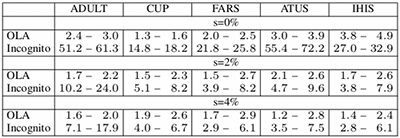It can be seen that Flash outperforms the other algorithms for all configurations on all datasets. The largest performance gain in comparison to the Incognito algorithm exists for a 0% suppression rate with a factor of more than 70 for ATUS (k = 8). Flash especially outperforms OLA on the two largest datasets at a 0% suppression rate, with a factor of about 4 for ATUS (k = 8) and a factor of about 5 for IHIS (k = 10). A more detailed comparison for selected configurations is shown in the following figure: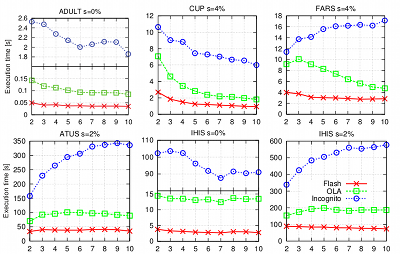In contrast to previous approaches, Flash offers stable execution times. This means that the execution time of the algorithm does not depend on the order of the columns in the input dataset or the algorithm that is used to build the generalization lattice. The following figure shows a comparison of the estimated execution times of our algorithm and OLA (including a stable variant) for all possible permutations of the input datasets: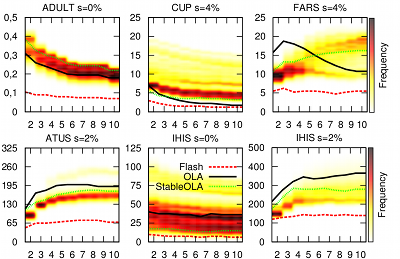While the estimated execution times of OLA vary greatly, our algorithm offers stable execution times and outperforms OLA and its stable variant in all cases. Flash even outperforms OLA when processing the best possible permutation in 114 out of 135 cases. Another systematic comparison of Flash with previous algorithms is provided by AnonBench.